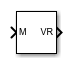Main Content

# MATLAB to VR Coordinates

Convert MATLAB coordinates to VR coordinates

• Library:
• Simulink 3D Animation / Utilities

•## Description

The MATLAB to VR Coordinates block converts a point with coordinates in the MATLAB® coordinate system to the VRML coordinate system.

The following relation holds between the two coordinate systems:

[xm, ym, zm] = [xv, zv, -yv]

where MATLAB coordinates are denoted with the m subscript and Virtual World coordinates are denoted with the v subscript. For more information on the two coordinate systems, see Virtual World Coordinate System.

## Ports

### Input

expand all

Coordinates of a point in MATLAB notation, specified as a 3-element row vector.

Data Types: `single` | `double`

### Output

expand all

Coordinates of a point in VRML notation, returned as a 3-element row vector.

Data Types: `single` | `double`

## See Also

### Topics

Introduced in R2019a

## SupportGet trial now